# The Drift Diffusion Model in Javascript

A lot of times when we're modeling decision making, we model decisions with something like a softmax function. This, however, doesn't take into account the computational difficulty in making decisions. How do we model differing reaction times in making a decision?

Enter the drift diffusion model. The drift diffusion model basically models decisions as a Gaussian random walk with a stopping time. Here I've included a tool that will allow you to play around with a drift diffusion model.

Threshold:

Drift:

Simulations:

Maxtime:

# Experiments with Latent Dirichlet Allocation

In a couple of my previous posts I talked about using clustering colors with k-means and counting clusters with EM. This kind of clustering is fairly straightforward, as you have some notion of distance between points to judge similarity. But what if you wanted to cluster text? How do you judge similarity there? (There are certain measures you could use, like the F-measure, which I’ll talk about in a later post.)

One way is to use Latent Dirichlet Allocation, which I first heard about while talking to a Statistics 133 GSI, and then later learned about while reading probabilistic models of cognition. Latent Dirichlet Allocation is a generative model that describes how text documents could be generated probabilistically from a mixture of topics, where each topic has a distribution over words. For each word in a document, a topic is sampled, from which a word is then sampled. This model gives us probabilities of documents, given topic distribution and words. But what’s more interesting here is learning about topics given the observed documents.

Here’s the plate notation view of LDA, which describes exactly how documents are generated: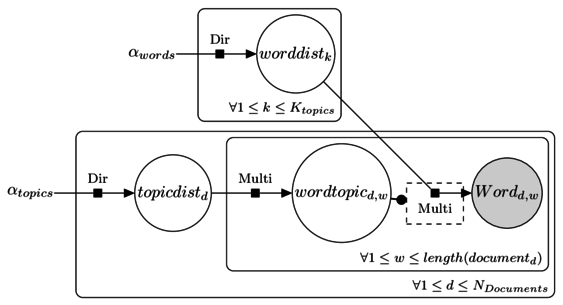# Counting clusters with mixture models and EM

I remember back when taking a Bayesian statistics course we were able to guess the number of subpopulations of fish based on a histogram of fish length measurements. Well, a few months later I totally forgot how we did this so I set out to relearn it. This problem in general is called “clustering”, where one finds “clusters” in the data. I’ve talked about clustering a bit before on my post on using k-means clustering to generate color themes from pictures. Here I’ll talk about clustering using mixture modeling and the EM algorithm and how we can use this model to get an idea of how many clusters are in our data set.

Take the (artificial) example below. It looks like there are populations of points: one broad circular one, and a denser diagonal one in the middle. How do we decide which of the two clusters points belong to, and even before that, how do we even decide that there are only two clusters?# Color theme generation from images using k-means

Note, this post more or less follows this post by Charles Leifer, except in less detail, and explained more poorly.

One of the top posts on the unixporn subreddit (SFW, really.) is this post that shows how a redditor generates color themes for his window manager from images using a script. He gets the code from Charles Leifer, who explains how the script works. Basically, the script detects the dominant colors in the image using k-means clustering.

As an exercise, I tried recreating the script in R. I didn’t exactly look at Charles’ code, but I knew the basic premise was that it uses k-means to generate a color palette.

I liked the idea of using R over Python because (a) as a statistics major I use R all the time and (b) there’s no other reason, R’s just fairly nice to work with.

# Color spaces

k-means performs differently depending on how you represent colors. A common color space to use is RGB, which represents colors by their red, green, and blue components. I found that representing colors in this manner tended to result in points along the diagonal. This happens since images usually have many shades of the same color, so, if you have $(r, g, b)$ you also tend to have $(r+10, g+10, b+10)$. This results in clusters having a sort of elongated shape, which isn’t that great for k-means since it seems better at picking out more “round” clusters. There is often a lot of correlation between dimensions. Maybe I’m not making a lot of sense here, suffice to say I wasn’t terribly pleased with the clusters I was getting.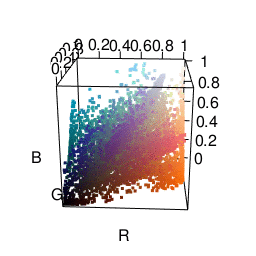The next color space I tried was HSV, which represents colors in terms of hue, saturation, and value. This actually got me some fairly satisfactory clusters. As you can see in the graphic below, it’s much easier to separate different colors. The only problem was that it made me want to put more weight on the “hue” dimension than the “saturation” or “value” dimensions. Many clusters ended up just being gray.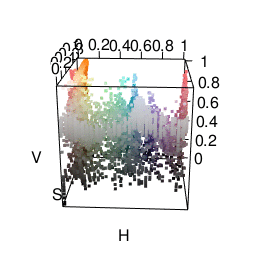One cool thing is that R already does HSV fairly easily using the rgb2hsv function.

I was most satisfied using LAB space. This represents colors with one “lightness” dimension and two color dimensions “A” and “B”. It was made to approximate human vision, and as you can see from the graphic below, distances between colors seem more meaningful. In fact, using Lab space is a recommended way of finding color difference. A good package for using this in R is the colorspace package.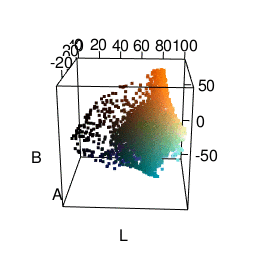## k-means

Another nice thing about R is that it has its own kmeans function built in. I actually tried writing my own, which looks like this:

## Do k-Means
## It tends to lose some k values
kMeans <- function(k, X, iter = 5) {
## Assign random membership
membership <<- sample(1:k, size=nrow(X), replace=TRUE)

for(i in 1:iter) {
mus <<- tapply(1:nrow(X), membership, function(x) colMeans(X[x,,drop=FALSE]), simplify=FALSE)
dd <<- do.call(cbind, lapply(mus, function(mu) rowSums((matrix(mu, byrow=TRUE,nrow=nrow(X), ncol=length(mu)) - X)^2)))
newmembership <<- apply(dd, 1, which.min)
if(all(newmembership == membership))
break
membership <<- newmembership
}
list(mus = mus, membership = membership)
}

Unfortunately it’s liable to return fewer clusters than requested. I think what’s going on is that in some iterations no points are closest to a specific cluster, so it’s lost. Perhaps there’s a bug somewhere I need to fix. Anyway, I ended up using the kmeans function instead.

It may be interesting to use other clustering techniques. I use k-means here only because it’s relatively easy to use. However, I would like to try distribution-based clustering at some point.

## From clusters to palette

Going from a list of colors to a palette configuration also requires some special thought. Given a list of colors like below, how do we pick which ones become a foreground color, background color, etc?We’d like our foreground and background colors in xterm to be chosen so they have a lot of contrast. This is where Lab space is very convenient: color difference is calculated just using Euclidean distance. We can then use the dist function in R to directly create a distance matrix from our color clusters represented in LAB form.

The way I ended up generating a palette was doing the following:

1. For the background color, take the first cluster (which is most represented color in the image). Then find the most different color for the foreground color.
2. With remaining colors, find pairs of colors that are very similar. The first in the pair gets set as something like color0 while the second gets set to color8.

Doing this, we end up getting a fairly nice palette from an image.

Here’s an example image used: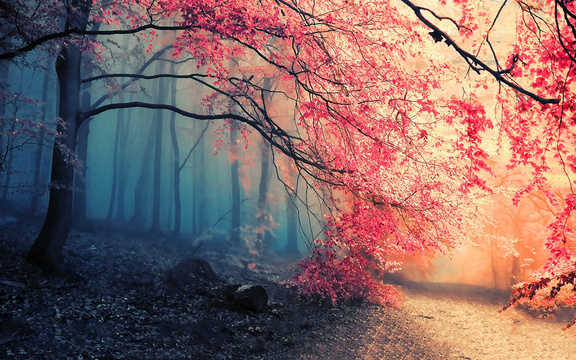And how it ends up looking in xterm: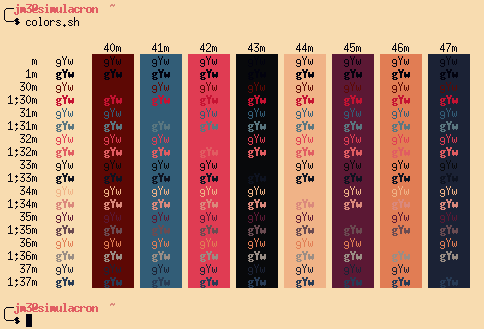## The actual code

Here’s all the actual code I used. It’s an Rscript that takes in a JPEG file as an argument and creates and xterm palette.